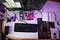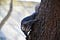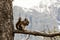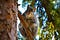# Generators in Python: Fundamentals for Data Scientists

## Understand the basics with a concrete example!Photo by Oskar Yildiz on Unsplash
`# Simple Generatordef my_simple_generator(): k = 0 yield k k = 1 yield k k = 2 yield kmy_numbers = my_simple_generator()print(next(my_numbers))print(next(my_numbers))print(next(my_numbers))# Below call raises StopIteration as generator is fully iterated# print(next(my_numbers))# Defining Generators with Loopdef my_generator_with_loop(my_str): length = len(my_str) for k in range(length):  yield my_str[k]my_text = my_generator_with_loop("Coding")for char in my_text: print(char)# Defining Generators with Expressionsmy_generator_expression = (number**2 for number in range(4))print (sum(my_generator_expression))# Defining Generator Pipelinemy_generator_01 = (number**2 for number in range(40))my_generator_02 = (number-5 for number in my_generator_01)print(sum(my_generator_02))`Photo by alex lauzon on Unsplash

# Defining Generator Functions

`# Simple Generatordef my_simple_generator(): k = 0 yield kk = 1 yield kk = 2 yield kmy_numbers = my_simple_generator()print(next(my_numbers))print(next(my_numbers))print(next(my_numbers))# Below call raises StopIteration as generator is fully iterated# print(next(my_numbers))#Output012`Photo by Norja V on Unsplash

# Defining Generators with a Loop

`# Defining Generators with Loopdef my_generator_with_loop(my_str): length = len(my_str) for k in range(length):  yield my_str[k]my_text = my_generator_with_loop("Coding")for char in my_text: print(char)#OutputCoding`

# Defining Generators With Generator Expressions

`# Defining Generators with Expressionsmy_generator_expression = (number**2 for number in range(4))print (sum(my_generator_expression))#Output14`

# Defining Generator Pipelines

`# Defining Generator Pipelinemy_generator_01 = (number**2 for number in range(40))my_generator_02 = (number-5 for number in my_generator_01)print(sum(my_generator_02))#Output20340`Photo by alex lauzon on Unsplash

# Conclusion

Machine Learning and Data Science Enthusiasts, Automotive Engineer, Mechanical Engineer, https://www.linkedin.com/in/erdem-isbilen/

## More from Erdem Isbilen

Machine Learning and Data Science Enthusiasts, Automotive Engineer, Mechanical Engineer, https://www.linkedin.com/in/erdem-isbilen/